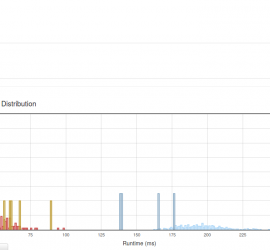# Daily Archives: April 10, 2015

## [leetcode] Valid Number — 用有限状态自动机解题1

Valid Number Validate if a given string is numeric. Some examples: “0” => true ” 0.1 ” => true “abc” => false “1 a” => false “2e10” => true Note: It is intended for the problem statement to be ambiguous. You should gather all requirements up front before implementing one. […]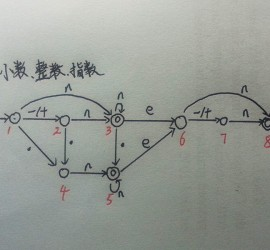## [leetcode] Unique Paths II

Unique Paths II Follow up for “Unique Paths”: Now consider if some obstacles are added to the grids. How many unique paths would there be? An obstacle and empty space is marked as 1 and 0 respectively in the grid. For example, There is one obstacle in the middle of […]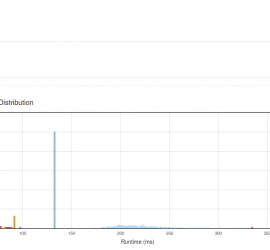## [leetcode] Unique Paths

Unique Paths A robot is located at the top-left corner of a m x n grid (marked ‘Start’ in the diagram below). The robot can only move either down or right at any point in time. The robot is trying to reach the bottom-right corner of the grid (marked ‘Finish’ […]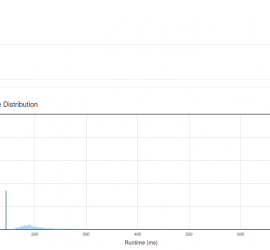## [leetcode] Rotate List

Rotate List Given a list, rotate the list to the right by k places, where k is non-negative. For example: Given 1->2->3->4->5->NULL and k = 2, return 4->5->1->2->3->NULL. 陷阱挺多，需要小心。 1. 如果链表为空或k==0，return head; 2. 如果k>链表长度count, k = k % count; /** * Definition for singly-linked list. * struct ListNode { * […]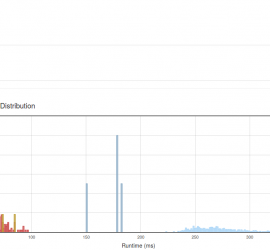## [leetcode] Permutation Sequence

Permutation Sequence The set [1,2,3,…,n] contains a total of n! unique permutations. By listing and labeling all of the permutations in order, We get the following sequence (ie, for n = 3): “123” “132” “213” “231” “312” “321” Given n and k, return the kth permutation sequence. Note: Given n […]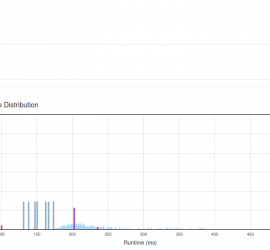## [leetcode] Spiral Matrix II

Spiral Matrix II Given an integer n, generate a square matrix filled with elements from 1 to n2 in spiral order. For example, Given n = 3, You should return the following matrix: [ [ 1, 2, 3 ], [ 8, 9, 4 ], [ 7, 6, 5 ] ] […]